2022: SklogWiki celebrates 15 years on-line

# Landau theory of phase transitions

In the Landau theory of phase transitions of Lev Davidovich Landau, the thermodynamic potential takes the form of a power series in the order parameter (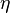$\eta$) (Eq. 143.1 of )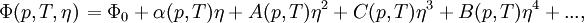$\left.\Phi(p, T, \eta)\right. = \Phi_0 + \alpha(p,T)\eta + A(p,T)\eta^2 + C(p,T)\eta^3 + B(p,T)\eta^4 + ...,$

where$p$ is the pressure and$T$ is the temperature. It is supposed that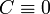$C \equiv 0$, leading to (Eq. 143.3)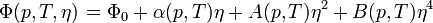$\left.\Phi(p, T, \eta)\right. = \Phi_0 + \alpha(p,T)\eta + A(p,T)\eta^2 + B(p,T)\eta^4$.

The the equilibrium state consistent with the external parameters$p$ and$T$ is a minimum of the Landau potential (often also called Landau free energy). This yields a prescription for determining the equilibrium value adopted by$\eta$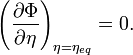$\left ( \frac{\partial\Phi}{\partial \eta}\right )_{\eta=\eta_{eq}} = 0.$

Taking into account very general symmetry properties of the underlying Hamiltonian one can set a priori the qualitative behaviour of the coefficients in the series. The value of those coefficients then very generally define the nature of the phase transition, which may be found to be either first or second order.

The Landau theory was the first successful attempt to highlight the universal features of phase transitions. However, the theory is known to fail for critical transitions of several types because of the neglect of fluctuations (i.e., the fact that the order parameter may fluctuate about the minima of$\eta$ and hence tunnel across free energy barriers separating two such minima.

## Illustration for the Ising model

For the Ising model one chooses the magnetization as order parameter. In the absence of external field, the underlying Hamiltonian is invariant to spin exchange. It must then follow that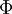$\Phi$ is also invariant to the sign of the magnetization. Hence, all odd coefficients of$\eta$ must vanish. For qualitative purposes, the free energy may be truncated to fourth order, thus: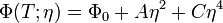$\Phi(T;\eta) = \Phi_0 + A\eta^2 + C\eta^4$

Equilibrium values for$\eta$ must then obey: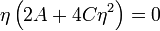$\eta \left (2A + 4C\eta^2 \right ) = 0$

This provides three possible candidates for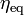$\eta_{\rm eq}$, mainly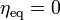$\eta_{\rm eq}=0$ and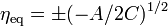$\eta_{\rm eq} = \pm (-A/2C)^{1/2}$. In order to rule out some candidates, we note that there must be only one possible solution above the Curie temperature,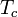$T_c$ (the system is then not magnetized), while two such solutions are expected below the Curie temperature. These conditions are fulfilled only if$A$ is an odd function of temperature, taking negative values below$T_c$ and positive values at high temperature. The simplest qualitative choice satisfying such conditions is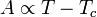$A\propto T-T_c$. The full behaviour then follows as:

#### Low temperature solution

At low temperature, there are two possible solutions ($\eta_{\rm eq}=0$ is here not a minimum but a maximum) corresponding to finite magnetization of opposite sign: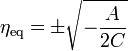$\eta_{\rm eq} = \pm \sqrt{-\frac{A}{2C}}$

The spontaneous emergence of finite magnetization from a Hamiltonian that is invariant to spin exchange is known as symmetry breaking.

As the critical point is approached,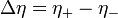$\Delta \eta = \eta_+ - \eta_-$ may be approximated as a power of the distance away from the critical point,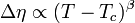$\Delta \eta \propto (T-T_c)^{\beta}$. From the solution of the Landau functional for the spontaneous magnetization, we find: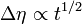$\Delta \eta \propto t^{1/2}$

Thus, according to the Landau's theory, the approach to criticality is governed by a power law with universal exponent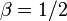$\beta=1/2$, regardless of any specific detail of the ferromagnetic fluid. To point out the universal behaviour of the exponent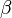$\beta$ is an achievement of Landau's theory. However, experimental studies have shown that the exponent is not quite 1/2, but rather, close to 1/3. The explanation of this discrepancy is the goal of renormalisation group theory of second order phase transitions.

#### High temperature solution

Above the Curie temperature there is only one possible solution corresponding to the disordered phase of zero magnetization,$\eta_{\rm eq}=0$ .

The qualitative nature of liquid--vapour phase transitions follow the same reasoning, with the order parameter now defined as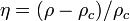$\eta = (\rho - \rho_c )/\rho_c$. The Ising model then usually takes the name of Lattice gas, with spins down standing for empty space, and spins up for molecules (or droplets). Such Lattice gas is a caricature of a compressible fluid along the coexistence line.

## Criticism

The Landau theory is phenomenological in nature. Nothing is known a priori about the coefficients of the series. Whereas this is a strong point in order to highlight universal features, it is clear that any useful assumption about the symmetry or vanishing of such coefficients requires a fair understanding of the transition beforehand